# High School Physics : Properties of Waves

## Example Questions

### Example Question #48 : Waves

After exercising, Jane takes her pulse. She realizes that her heart is beating rapidly, approximately four beats every second. What is the period of her elevated heart rate?

Possible Answers: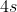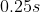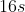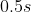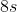Correct answer:Explanation:

When you see a relationship like "times every second" or "once per hour," these are hints you are looking at a frequency. Frequency is, effectively, how often something happens. If it happens four times per second, then we know how often it happens. The units "per second" are equivalent to Hertz.

The relationship between frequency and period is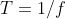.

Since our given frequency was four beats per second, or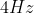, we can solve for the period.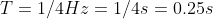This means that her heart beats once every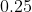seconds.

### Example Question #51 : Waves

A wave oscillates with a speed of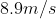and has a wavelength of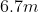. What is the frequency of the wave?

Possible Answers: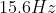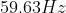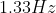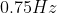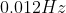Correct answer:Explanation:

The equation for velocity in terms of wavelength and frequency is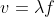.

We are given the velocity and the wavelength. Using these values, we can solve for the frequency.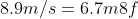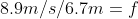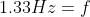### Example Question #52 : Waves

A wave with a constant velocity doubles its frequency. What happens to its wavelength?

Possible Answers:

The new wavelength will be 12  the old wavelength.

The new wavelength will also double.

There is insufficient information to solve.

The wavelengths will be the same.

Correct answer:

The new wavelength will be 12  the old wavelength.

Explanation:

The relationship between velocity, frequency, and wavelength is: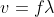In this case we're given a scenario where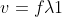and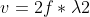. The velocities equal each other because the problem states it has a constant velocity. Therefore we can set these equations equal to each other: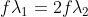Notice that the f's cancel out: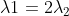Divide both sides by two: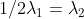### Example Question #53 : Waves

Two notes are played simultaneously. One of them has a period of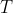and the other has a period of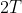. Which one has a longer wavelength?

Possible Answers:We need to know the frequency in order to solve

They have the same wavelength

We need to know the period in order to solve

Correct answer:Explanation:

The relationship between frequency and wavelength determines the velocity:The frequency is the inverse of the period. We can substitute this into the equation above.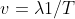In the question, both of the notes are played at the same time in the same location, so they both should have the same velocity. We can set the equation for each tone equal to each other.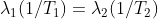We are told that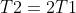. Substitute into our equation.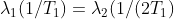We can cancel the period from each side of the equation, leaving the relationship between the two wavelengths.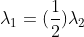The wavelength of the first wave is equal to half the wavelength of the second. This means that the wavelength for the tone with a longer period will have a longer wavelength as well.

### Example Question #54 : Waves

A wave has a frequency of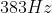. What is its period?

Possible Answers: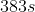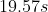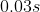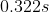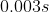Correct answer:Explanation:

The relationship between frequency and period is.

Plug in our given value: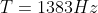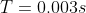### Example Question #55 : Waves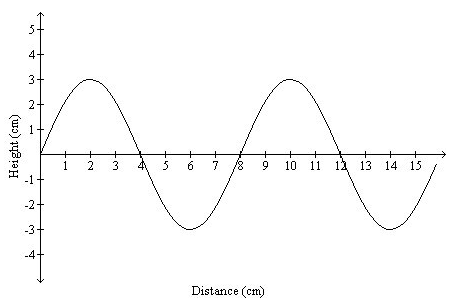What is the wavelength of the wave above?

Possible Answers: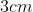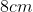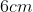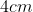Correct answer:Explanation:

The wavelength of a wave is defined as the distance from a point on the wave to the same point on the wave (crest to crest or trough to trough). The distance between peaks is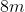.

### Example Question #56 : WavesWhat is the amplitude of the wave above?

Possible Answers:Correct answer:Explanation:

The amplitude of wave is defined as the distance the wave displaces from the equilibrium point.  In this case, the wave displacesfrom the axis (the equilibrium point).

### Example Question #57 : Waves

A student attaches one end of a Slinky to the top of a table. She holds the other end in her hand, stretches it to a length, and then moves it back and forth to send a wave down the Slinky. If she next moves her hand faster while keeping the length of the Slinky the same, how does the wavelength down the slinky change?

Possible Answers:

It decreases

It stays the same

It increases

Correct answer:

It decreases

Explanation:

The speed of the wave along the Slinky depends on the mass of the Slinky itself and the tension caused by stretching it. Since both of these things have not changed, the wave speed remains constant.

The wave speed is equal to the wavelength multiplied by the frequency.Since she is moving her hand faster, the frequency has increased. Since the velocity has not changed, an increase in the frequency would decrease the wavelength

### Example Question #58 : Waves

Resonance in a system, such as a string fixed at both ends, occurs when

Possible Answers:

It is oscillating in simple harmonic motion

Its frequency is less than the frequency of the external source

Its frequency is the same as the frequency of the external source

Its frequency is greater than the frequency of the external source

Correct answer:

Its frequency is the same as the frequency of the external source

Explanation:

The frequency at which standing waves are produced is known as the resonant frequencies. When two objects are brought near each other, and they both make standing waves at the same frequency, there is resonance in the system. For example, if you have two tuning forks of the same note, you can tap one and bring it close (but not touch) the other. Then if you silence the first tuning fork and listen, you will hear the second fork ringing as well because it vibrates at the same frequency.

### All High School Physics Resources